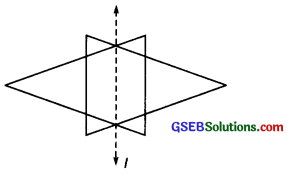# GSEB Solutions Class 6 Maths Chapter 13 Symmetry Ex 13.1

Gujarat Board GSEB Textbook Solutions Class 6 Maths Chapter 13 Symmetry Ex 13.1 Textbook Questions and Answers.

## Gujarat Board Textbook Solutions Class 6 Maths Chapter 13 Symmetry Ex 13.1

Question 1.
List any four symmetrical objects from your home or school.
Solution:
Following objects can be symmetrical objects:
(i) A pair of scissors
(ii) An electric bulb
(iii) A table fan
(iv) A blackboard

Question 2.
For the given figure, which one is the mirror line, l1 or l2?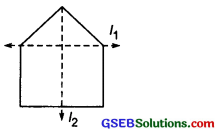Solution:
In the given figure, the line If is the mirror line.Question 3.
Identify the shapes given below. Check whether they are symmetric or not. Draw the line of symmetry as well.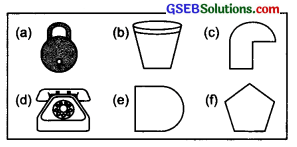Solution:
(a) The given figure is symmetric. Its line of symmetry is shown by the dotted line.(b) The given figure is symmetric. Its line of symmetry is shown by the dotted line.(c) The given figure is not symmetric.(d) The given figure is symmetric and its line of symmetry is shown by the dotted line.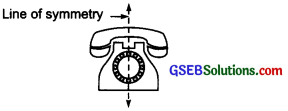(e) The given figure is symmetric and its line of symmetry is shown by the dotted line.(f) The given figure is symmetric and its line of symmetry is shown by the dotted line.Question 4.
Copy the following on a squared paper. A square paper is what you would have used in your arithmetic notebook in earlier classes. Then complete them such that the dotted line is the line of symmetry.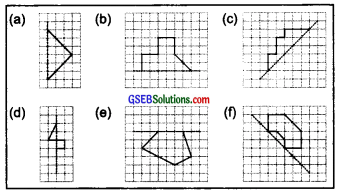Solution:
Considering the dotted line as the line of symmetry, we can complete the figures as follows:Question 5.
In the figure, l is the line of symmetry. Complete the diagram to make it symmetric.Solution:
The completed figure is given below: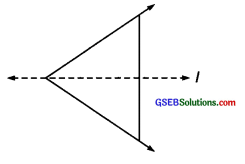Question 6.
In the figure, l is the line of symmetry. Draw the image of the triangle and complete the diagram so that it becomes symmetric.Solution:
The image of the triangle is shown in the figure given below.